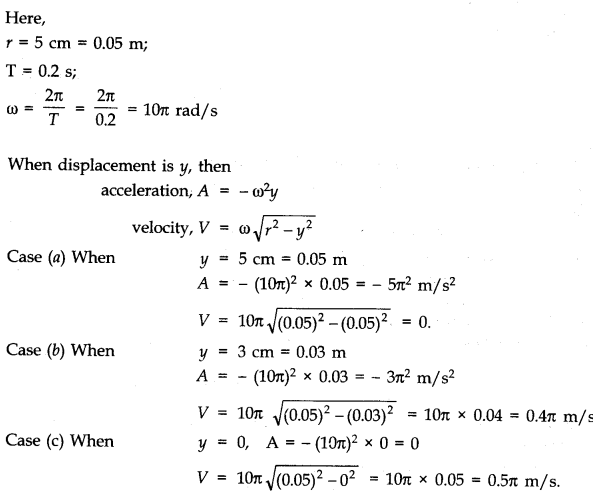# Simple Harmonic Motion Formula

Simple harmonic motion formula is used to obtain the position, velocity, acceleration, and time period of an object which is in simple harmonic motion. To recall, SHM or simple harmonic motion is one of the special periodic motion in which the restoring force is directly proportional to the displacement and it acts in the opposite direction where the displacement occurs.

### Formulas for Simple Harmonic Motion

• $$\begin{array}{l}Position \, (x)\,=\,A\,sin(\omega t\,+\, \phi)\end{array}$$
• $$\begin{array}{l}Velocity\; of \; SHM\, (v(t))\,=\, A\, \omega\, cos(\omega\,t\,+\,\phi)\end{array}$$
• $$\begin{array}{l}Acceleration\; of \; SHM\, (a(t))\,=\, \frac{d^{2}x}{dt^{2}}\,=\, -\omega^{2}\,x(t)\end{array}$$
• $$\begin{array}{l}Time\; Period \; of \; SHM\, (T)\,=\, 2\pi\sqrt{\frac{m}{k}}\end{array}$$

Note: In the above formulas, the meaning of each term are:

A = amplitude (m)

$$\begin{array}{l}\omega\, =\, angular\, frequency\end{array}$$
$$\begin{array}{l}\phi\,=\, phase \, shift\end{array}$$

### Example Questions from SHM

Example 1:

A ball attached to a string is in simple harmonic motion. Find the position of that ball at t= 2 seconds if the amplitude of that ball’s motion is 0.080 m, its angular frequency is 7.07 radians/second, and the phase shift is 0 radians.

Solution:

Given,

A= 0.080 m

t= 2 seconds

$$\begin{array}{l}\omega= 7.07\,radians/second\end{array}$$
$$\begin{array}{l}\phi=0.0\, radians\end{array}$$

From the positions formula,

$$\begin{array}{l}Position \, (x)\,=\,A\,sin(\omega t\,+\, \phi)\end{array}$$

Now by putting the values in the above formula, the following is obtained-

$$\begin{array}{l}x\,=\,(0.080\,m)\, sin[(7.07\, radians/second)(2 second)\,+\, 0\, radians]\end{array}$$

By solving this, the position of the object at t=2 second is obtained.

X = 0.080 m.

Example 2:

A body is in simple harmonic motion is having an amplitude of 5 cm and a period of 0.2 s. Calculate the acceleration and the velocity of the body when the displacement is (a) 5 cm (b) 3 cm (c) 0 cm.

Solution:Stay tuned with BYJU’S to get more physics formulas, derivations, and other preparation materials.